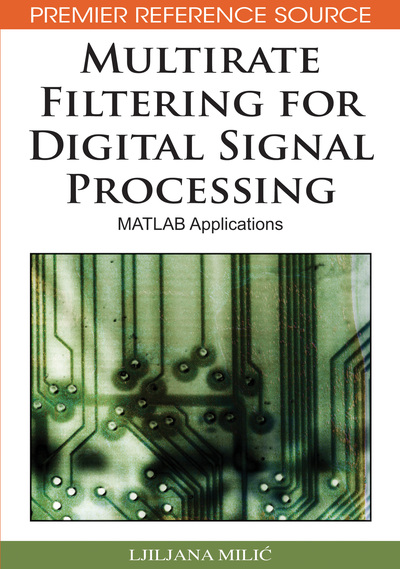# Complementary Filter Pairs

Ljiljana Milic (Mihajlo Pupin Institute, Serbia)
DOI: 10.4018/978-1-60566-178-0.ch008
Available
\$33.75
List Price: \$37.50
10% Discount:-\$3.75
TOTAL SAVINGS: \$3.75

## Abstract

Digital filters with complementary characteristics find many applications in practice. In this chapter, we concentrate on the properties and construction of complementary filters and filter pairs. An important application of complementary property is deriving a new transfer function from the existing one. A highpass filter can be obtained as a complement of the lowpass filter, and also a bandstop filter can be considered as a complement of the bandpass filter. Complementary lowpass/highpass and bandpass/bandstop filter pairs are popular because of very attractive implementations. Namely, the two complementary filters in the pair are implemented at the cost of a single one. Complementary filter pairs, usually lowpass/highpass filter pairs, are widely used whenever there is a need to split the signal into two adjacent subbands and reconstruct it after some processing performed in the subbands. They are used as basic building blocks in constructing analysis and synthesis multichannel filter banks. Moreover, the complementary filter pairs are used in constructing low sensitivity complex filtering structures. In some applications, such as signal analysis, the complementary filter pairs are used to separate a signal into two bands, and the filtered signals are processed without need to reconstruct it. Another application is digital audio where the signal is separated into two (three) bands resulting in the signals that are feed inside two (three) loudspeakers. In this chapter, at the beginning we introduce the basic definitions of the complementary properties that will be used through the chapter. We use then the complementary properties to construct FIR and IIR highpass filters from the existing lowpass filters. In the sequel, we consider the analysis and synthesis filter pairs. We present the design and efficient implementations of FIR and IIR complementary filter pairs. Chapter concludes with MATLAB Exercises for individual study.
Chapter Preview
Top

## Delay-Complementary Filter Pairs

A filter pair [H(z), HC(z)] is said to be delay-complementary, or strictly-complementary if the transfer functions H(z) and HC(z) add up to a delay. (1) Here, K > 0 is a constant, typically K = 1; and n0 is a nonnegative integer.

### All-Pass Complementary Filter Pairs

A filter pair [H(z), HC(z)] is an all-pass-complementary filter pair if the sum of H(z) and HC(z) satisfies. (2) where A(z) is an all-pass transfer function.

Top

## Power-Complementary Filter Pairs

A filter pair [H(z), HC(z)] is a power-complementary filter pair if the sum of the squares of their magnitude responses satisfies

(3) where K > 0 is a constant, typically K = 1. For this pair, the angular frequency ω = ωc, where (4) is the crossover angular frequency. At this angular frequency, the gain responses of both filters are approximately 3 dB below their maximum values.

## Complete Chapter List

Search this Book:
Reset